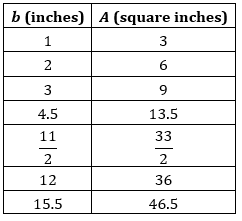# Week 8 Day 1 Opener

The table shows the relationship between the base length, b, and the area, A, of some parallelograms. All the parallelograms have the same height. Base length is measured in inches, and area is measured in square inches.Decide whether each equation could represent the relationship between b and A. a) b = 3A b) b = A/3 c) A = b/3 d) A = 3b

If we know the base, which equation would make it easier to find the area? Why?

If we know the area, which equation would make it easier to find the base? Why?